JEE  >  JEE Advanced Practice Test- 11

# JEE Advanced Practice Test- 11 - JEE

Test Description

## 54 Questions MCQ Test JEE Main & Advanced Mock Test Series - JEE Advanced Practice Test- 11

JEE Advanced Practice Test- 11 for JEE 2023 is part of JEE Main & Advanced Mock Test Series preparation. The JEE Advanced Practice Test- 11 questions and answers have been prepared according to the JEE exam syllabus.The JEE Advanced Practice Test- 11 MCQs are made for JEE 2023 Exam. Find important definitions, questions, notes, meanings, examples, exercises, MCQs and online tests for JEE Advanced Practice Test- 11 below.
 1 Crore+ students have signed up on EduRev. Have you?
JEE Advanced Practice Test- 11 - Question 1

### This section contains 4 multiple choice questions numbered 1 to 4. Each question has 4 choices (A), (B), (C) and (D), out of which ONLY ONE is correct A particle is kept at rest at the top of a sphere of diameter 42 m. When disturbed slightly, it slides down. At what height h from the bottom, the particle will leave the sphere

JEE Advanced Practice Test- 11 - Question 2

### A source is moving on a circle of radius 3 m with constant angular velocity ω = 5 rad/s. If the observer is at a distance 5 m from the centre of circle, the time interval between maximum and minimum frequency received by the observer is

Detailed Solution for JEE Advanced Practice Test- 11 - Question 2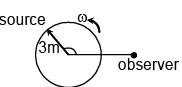JEE Advanced Practice Test- 11 - Question 3

### In the cyclic process 1-2-3-4 shown in figure for a monoatomic gas, thevolume of the gas in process 1.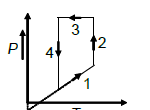Detailed Solution for JEE Advanced Practice Test- 11 - Question 3

In a P-V diagram clockwise cyclic process means positive work is done. Here, with non zero anti clockwise area negative work is being done.
W = P∆V
∆V is negative, since, P is positive.
So, the volume decreases.

JEE Advanced Practice Test- 11 - Question 4

A capacitor is charged to a potential of V0. It is connected with an inductor through a switch S. The switch is closed at time t = 0. Which of the following statement is correct.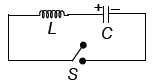*Multiple options can be correct
JEE Advanced Practice Test- 11 - Question 5

This section contains 3 multiple choice questions. Each question has 4 choices (A), (B), (C) and (D) for its answer, out of which ONE OR MORE is/are correct

A disc of radius R rolls on a horizontal surface with linear velocity V and angular velocity ω. There is a point P on circumference of disc at angle θ with upward vertical diameter measured anticlockwise (see figure), which has a vertical velocity. Here θ is equal to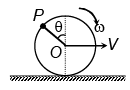*Multiple options can be correct
JEE Advanced Practice Test- 11 - Question 6

A source of sound moves along a circle of radius 2 m with constant angular velocity 40 rad/s. Frequency of the source is 300 Hz. A detector is kept at some distance from the circle in the same plane of the circle (as shown in figure). Which of the following is not the possible value of frequency registered by the detector? (Speed of sound = 320 m/s)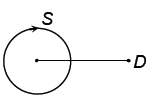*Multiple options can be correct
JEE Advanced Practice Test- 11 - Question 7

An electron in H-atom jumps from second excited state to first excited state and then from first excited to ground state. Let the ratio of wavelength, momentum and energy of photons emitted in these two cases be a, b and c respectively. Then

JEE Advanced Practice Test- 11 - Question 8

This section contains 2 paragraphs. Based upon one of paragraph 2 multiple choice questions and based on the other paragraphs 3 multiple choice questions have to be answered. Each of these questions has four choices (A), (B), (C) and (D) out of which ONLY ONE is correct

Paragraph for Question Nos. 10 and 11

Four satellites A, B, C and D are orbiting around a spherical planet of uniform density. The orbits of all the satellites are coplanar. Orbits of satellites A and B are circular where radius of orbit A is greater than diameter of orbit B. Orbit C is touching orbits A and B. While orbit D is touching orbit A but its nearest distance from the planet is least among all four orbits. All the satellites have same mass and these satellite can be considered as particles. There is no gravitational force between the satellite and also there is no chance of collision between the satellites. Consider gravitational potential energy to be zero under infinite separation.

In which orbit can the gravitational potential energy have least possible value?

JEE Advanced Practice Test- 11 - Question 9

Four satellites A, B, C and D are orbiting around a spherical planet of uniform density. The orbits of all the satellites are coplanar. Orbits of satellites A and B are circular where radius of orbit A is greater than diameter of orbit B. Orbit C is touching orbits A and B. While orbit D is touching orbit A but its nearest distance from the planet is least among all four orbits. All the satellites have same mass and these satellite can be considered as particles. There is no gravitational force between the satellite and also there is no chance of collision between the satellites. Consider gravitational potential energy to be zero under infinite separation.

In which orbit does the angular momentum have largest magnitude?

JEE Advanced Practice Test- 11 - Question 10

Paragraph for Question Nos. 12 and 14

A thin non-conducting ring of mass m, radius a carrying a charge q can rotate freely about its own axis which is vertical. At the initial moment the ring was at rest in horizontal position and no magnetic field was present. At instant t = 0 A uniform magnetic field is switched on which is vertically downward and increases with time according to the law B = B0t. Neglecting magnetism induced due to rotational motion of ring.

The magnitude of induced emf will be

JEE Advanced Practice Test- 11 - Question 11

A thin non-conducting ring of mass m, radius a carrying a charge q can rotate freely about its own axis which is vertical. At the initial moment the ring was at rest in horizontal position and no magnetic field was present. At instant t = 0 A uniform magnetic field is switched on which is vertically downward and increases with time according to the law B = B0t. Neglecting magnetism induced due to rotational motion of ring.

The magnitude of an electric field on the surface of the ring is

JEE Advanced Practice Test- 11 - Question 12

A thin non-conducting ring of mass m, radius a carrying a charge q can rotate freely about its own axis which is vertical. At the initial moment the ring was at rest in horizontal position and no magnetic field was present. At instant t = 0 A uniform magnetic field is switched on which is vertically downward and increases with time according to the law B = B0t. Neglecting magnetism induced due to rotational motion of ring.

Find instantaneous power developed by electric force acting on the ring at t = 1 sec.

JEE Advanced Practice Test- 11 - Question 13

This section contains 2 questions. Each question contains statements given in two columns, which have to be matched. The statements in Column I are labelled A, B, C and D, while the statements in Column II are labelled p, q, r, s and t. Any given statement in Column I can have correct matching with ONE OR MORE statement(s) in Column II. The appropriate bubbles corresponding to the answers to these questions have to be darkened as illustrated in the following example:

If the correct matches are A – p, s and t; B – q and r; C – p and q; and D – s and t; then the correct darkening of bubbles will look like the following:

Figure shows a set-up to perform Young’s double slit experiment. A monochromatic source of light is placed at S. S1 and S2 act as coherent sources and interference pattern is obtained on the screen. Match Column-I with Column-II in regard to interference in Young’s double slit
experiment: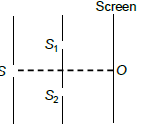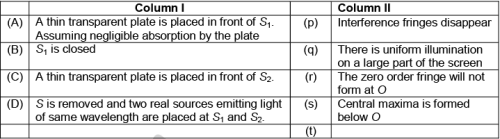JEE Advanced Practice Test- 11 - Question 14

Referring to the given circuit, match Column-I with Column-II.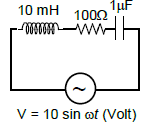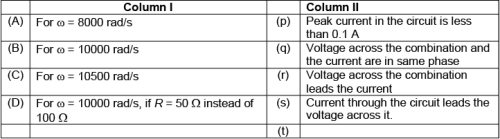*Answer can only contain numeric values
JEE Advanced Practice Test- 11 - Question 15

This section contains 5 questions. Each question, when worked out will result in one integer from 0 to 9 (both inclusive).

The lengths of sides of a cuboid are a, 2a and 3a. If the relative percentage error in the
measurement of a is 1%, then find the relative percentage error in the measurement of volume of cube (in percentage)….

*Answer can only contain numeric values
JEE Advanced Practice Test- 11 - Question 16

For the arrangement shown in figure, the masses of the blocks are mA = 2 kg, mB = 1 kg, mC = 1 kg. Find the frictional force between the blocks A and B (in Newton) (coefficient of friction between any two surfaces is 0.5)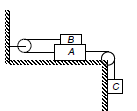*Answer can only contain numeric values
JEE Advanced Practice Test- 11 - Question 17

Suppose potential energy between particle A and B at separation r is given by U = k ln r, where k is constant. For such hypothetical system, find the ratio of energy difference between energy
levels (n = 1 and n = 2) and (n = 2 and n = 4) (The energy of a particle in nth level is –13.6/n2)

*Answer can only contain numeric values
JEE Advanced Practice Test- 11 - Question 18

A uniform disc of radius R having charge Q distributed uniformly all over its surface is placed on a smooth horizontal surface. A magnetic field, B = kxt2, where k is a constant, x is the distance (in metre) from the centre of the disc and t is the time (in second), is switched on perpendicular to the plane of the disc. Find the torque (in N-m) acting on the disc after 15 sec. (Take 4kQ = 1 S.I.unit and R = 1m) is

*Answer can only contain numeric values
JEE Advanced Practice Test- 11 - Question 19

The hydrogen atom in its ground state are excited by means of monochromatic radiation of
wavelength 1023 Å. Find the number of lines which can be seen in the resulting spectrum…

JEE Advanced Practice Test- 11 - Question 20

Potash alum contains the metals like potassium and aluminium. What are the metal ions present in chrome alum?

Detailed Solution for JEE Advanced Practice Test- 11 - Question 20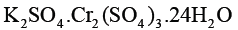JEE Advanced Practice Test- 11 - Question 21

For a gaseous reaction, the rate of reaction is expressed in terms of dp/dt instead of dc/dt or dn/dt where C is concentration and n is the number of moles. Hence, the relation between expression is:

Detailed Solution for JEE Advanced Practice Test- 11 - Question 21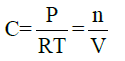JEE Advanced Practice Test- 11 - Question 22

An excited electron of H-atoms emits of photon of wavelength λ and returns in the ground state, the principal quantum number of excited state is given by:

Detailed Solution for JEE Advanced Practice Test- 11 - Question 22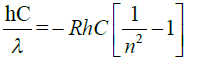JEE Advanced Practice Test- 11 - Question 23

One mole of a monatomic real gas satisfies the equation p (V - b) = RT where b is a constant. The relationship of interatomic potential V (r ) and interatomic distance r for the gas is given by

Detailed Solution for JEE Advanced Practice Test- 11 - Question 23

Here attraction is zero , so potential energy can’t be less than 0

*Multiple options can be correct
JEE Advanced Practice Test- 11 - Question 24

This section contains 3 multiple choice questions. Each question has 4 choices (A), (B), (C) and (D) for its answer, out of which ONE OR MORE is/are correct

Identify the correct relationship:

Detailed Solution for JEE Advanced Practice Test- 11 - Question 24

Ni2+ complex becomes 3d8 with all e- paired.

*Multiple options can be correct
JEE Advanced Practice Test- 11 - Question 25

Identify the chiral molecule/molecules:

Detailed Solution for JEE Advanced Practice Test- 11 - Question 25

Chiral molecules do not have plane of symmetry

*Multiple options can be correct
JEE Advanced Practice Test- 11 - Question 26

The correct statement/statements is/are:

JEE Advanced Practice Test- 11 - Question 27

This section contains 2 paragraphs. Based upon one of paragraph 2 multiple choice questions and based on the other paragraphs 3 multiple choice questions have to be answered. Each of these questions has four choices (A), (B), (C) and (D) out of which ONLY ONE is correct

Paragraph for Question Nos. 10 and 11

The cloud consist of charged particles of water dispersed in air. Some of them are +vely charged and other are -vely charged. When +vely charged clouds come closer they have lightening and thundering whereas - ve and +ve charged colloids come closer they cause heavy rain due to the aggregation of minute particles. It is possible to cause artificial rain by throwing electrified sand or AgI from aeroplane and thus coagulation the mist hanging in air.

When excess of 3 AgNO is treated withKI solution, AgI forms.

Detailed Solution for JEE Advanced Practice Test- 11 - Question 27

+vely charged colloid and –vely charged solution

JEE Advanced Practice Test- 11 - Question 28

The cloud consist of charged particles of water dispersed in air. Some of them are +vely charged and other are -vely charged. When +vely charged clouds come closer they have lightening and thundering whereas - ve and +ve charged colloids come closer they cause heavy rain due to the aggregation of minute particles. It is possible to cause artificial rain by throwing electrified sand or AgI from aeroplane and thus coagulation the mist hanging in air.

Electrical chimney are made on the principal of

Detailed Solution for JEE Advanced Practice Test- 11 - Question 28

Neutralisation of charge of colloidal particle.

JEE Advanced Practice Test- 11 - Question 29

Paragraph for Question Nos. 12 and 14

Compound (A) , which can be distilled in high vacuum, gives positive test with  Br2 / H2O , but does not react with Nametal in ethereal solution, but compound (B) and (C) gives  H2 (g) with sodium metal. Compound (A) undergoes following sequence of reactions-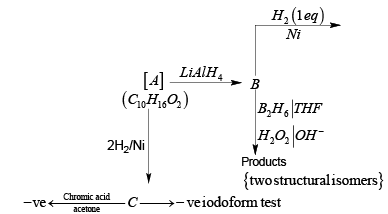Compound ' B' should be:

JEE Advanced Practice Test- 11 - Question 30

Compound (A) , which can be distilled in high vacuum, gives positive test with  Br2 / H2O , but does not react with Nametal in ethereal solution, but compound (B) and (C) gives  H2 (g) with sodium metal. Compound (A) undergoes following sequence of reactions-Compound ' A' should be:

Detailed Solution for JEE Advanced Practice Test- 11 - Question 30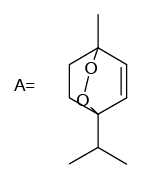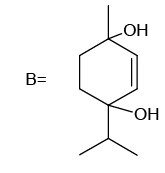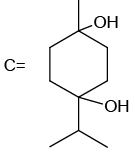JEE Advanced Practice Test- 11 - Question 31

Compound (A) , which can be distilled in high vacuum, gives positive test with  Br2 / H2O , but does not react with Nametal in ethereal solution, but compound (B) and (C) gives  H2 (g) with sodium metal. Compound (A) undergoes following sequence of reactions-Compound 'C '

JEE Advanced Practice Test- 11 - Question 32

This section contains 2 questions. Each question contains statements given in two columns, which have to be matched. The statements in Column I are labelled A, B, C and D, while the statements in Column II are labelled p, q, r, s and t. Any given statement in Column I can have correct matching with ONE OR MORE statement(s) in Column II. The appropriate bubbles corresponding to the answers to these questions have to be darkened as
illustrated in the following example:
If the correct matches are A – p, s and t; B – q and r; C – p and q; and D – s
and t; then the correct darkening of bubbles will look like the following:

Match the following metal given in column-I with the appropriate metal exaction process listed
with column- II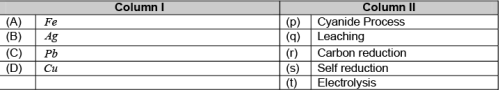JEE Advanced Practice Test- 11 - Question 33

This section contains 2 questions. Each question contains statements given in two columns, which have to be matched. The statements in Column I are labelled A, B, C and D, while the statements in Column II are labelled p, q, r, s and t. Any given statement in Column I can have correct matching with ONE OR MORE statement(s) in Column II. The appropriate bubbles corresponding to the answers to these questions have to be darkened as
illustrated in the following example:
If the correct matches are A – p, s and t; B – q and r; C – p and q; and D – s
and t; then the correct darkening of bubbles will look like the following:

Match the following metal given in column-I with the appropriate metal exaction process listed
with column- II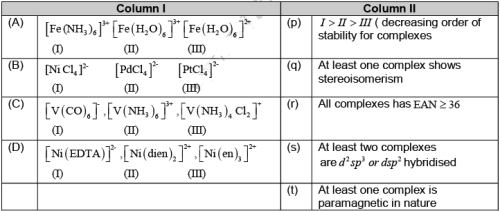*Answer can only contain numeric values
JEE Advanced Practice Test- 11 - Question 34

This section contains 5 questions. Each question, when worked out will result in one integer from 0 to 9 (both inclusive).

A saturated solution of spraingly soluble salt MCl2 has a vapour pressure of 31.78 mm of Hg at 300C while pure water exerts a pressure of 31.82 mm of Hg at the same temperature. The
solubility product is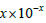. The value of x is

Detailed Solution for JEE Advanced Practice Test- 11 - Question 34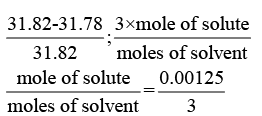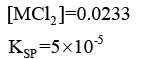*Answer can only contain numeric values
JEE Advanced Practice Test- 11 - Question 35

The number of unpaired e- in first excited state of O2 is

Detailed Solution for JEE Advanced Practice Test- 11 - Question 35

in excited state it is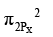*Answer can only contain numeric values
JEE Advanced Practice Test- 11 - Question 36

The number of geometrical isomers of the octahedral complex of the type [Mabcdef] is n x 3. . The value of n is

Detailed Solution for JEE Advanced Practice Test- 11 - Question 36

Total 15 gemetrical isomers

*Answer can only contain numeric values
JEE Advanced Practice Test- 11 - Question 37

How many double bond equivalents does amoxicillin (shown below) possesses?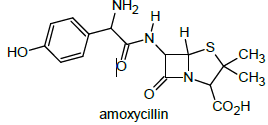JEE Advanced Practice Test- 11 - Question 38

Let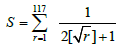where [.] denotes the greatest integer function. The value of S is

Detailed Solution for JEE Advanced Practice Test- 11 - Question 38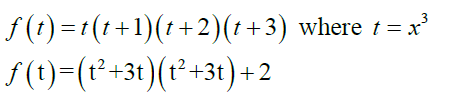Usemaxima minima concept

JEE Advanced Practice Test- 11 - Question 39

Minimum value of function f (x) = x3 (x3 +1)(x3 + 2)(x3 + 3) : x ∈ R, is

JEE Advanced Practice Test- 11 - Question 40

The hands of a clock has lengths 3 & 4 units. The distance between their tips when the distanceis increasing most rapidly is

JEE Advanced Practice Test- 11 - Question 41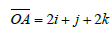is rotated about O (origin) by 90° such that it crosses the positive y axis during the rotation. If A goes to B then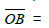*Multiple options can be correct
JEE Advanced Practice Test- 11 - Question 42

This section contains 3 multiple choice questions. Each question has 4 choices (A), (B), (C) and (D) for its answer, out of which ONE OR MORE is/are correct

In ΔABC, if A = 450, B = 750 then

*Multiple options can be correct
JEE Advanced Practice Test- 11 - Question 43

Equation of plane through A(1, 0, 0), B(0,1, 0) and making an angle π/4with the plane x + y = 3 canbe

*Multiple options can be correct
JEE Advanced Practice Test- 11 - Question 44

If the area bounded by the curve y = x - x2 and the line y = mx equals 9/2. Then the value of q m is

Detailed Solution for JEE Advanced Practice Test- 11 - Question 44

use graph

JEE Advanced Practice Test- 11 - Question 45

This section contains 2 paragraphs. Based upon one of paragraph 2 multiple choice questions and based on the other paragraphs 3 multiple choice questions have to be answered. Each of these questions has four choices (A), (B), (C) and (D) out of which ONLY ONE is correct

A box has 10 coins. Five have heads on both sides. Three have tails on both sides; two are fair. A coin is chosen at random and tossed.

Detailed Solution for JEE Advanced Practice Test- 11 - Question 45

JEE Advanced Practice Test- 11 - Question 46

This section contains 2 paragraphs. Based upon one of paragraph 2 multiple choice questions and based on the other paragraphs 3 multiple choice questions have to be answered. Each of these questions has four choices (A), (B), (C) and (D) out of which ONLY ONE is correct

A box has 10 coins. Five have heads on both sides. Three have tails on both sides; two are fair. A coin is chosen at random and tossed.

Probability that the coin is fair given that head has appeared is

JEE Advanced Practice Test- 11 - Question 47

ABC is a triangle. Complex numbers z1 , z2 , z3 are represented by A, B, C respectively. Which are in anticlock wise order. let |z1|=|z2|=|z3| and arg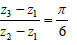Detailed Solution for JEE Advanced Practice Test- 11 - Question 47

Use rotation of complex numbers

JEE Advanced Practice Test- 11 - Question 48

Orthocentre H of ΔABC denotes the complex no.

Detailed Solution for JEE Advanced Practice Test- 11 - Question 48

Use rotation of complex numbers.

JEE Advanced Practice Test- 11 - Question 49

If T(z) is any point such that HBTC is a parallelogram then | z - z1 |=

Detailed Solution for JEE Advanced Practice Test- 11 - Question 49

Use rotation of complex numbers.

JEE Advanced Practice Test- 11 - Question 50

This section contains 2 questions. Each question contains statements given in two columns, which have to be matched. The statements in Column I are labelled A, B, C and D, while the statements in Column II are labelled p, q, r, s and t. Any given statement in Column I can have correct matching with ONE OR MORE statement(s) in Column II. The appropriate bubbles corresponding to the answers to these questions have to be darkened as
illustrated in the following example:
If the correct matches are A – p, s and t; B – q and r; C – p and q; and D – s
and t; then the correct darkening of bubbles will look like the following: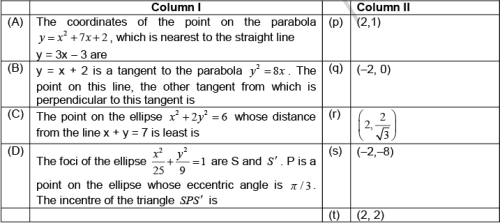JEE Advanced Practice Test- 11 - Question 51

This section contains 2 questions. Each question contains statements given in two columns, which have to be matched. The statements in Column I are labelled A, B, C and D, while the statements in Column II are labelled p, q, r, s and t. Any given statement in Column I can have correct matching with ONE OR MORE statement(s) in Column II. The appropriate bubbles corresponding to the answers to these questions have to be darkened as
illustrated in the following example:
If the correct matches are A – p, s and t; B – q and r; C – p and q; and D – s
and t; then the correct darkening of bubbles will look like the following:*Answer can only contain numeric values
JEE Advanced Practice Test- 11 - Question 52

This section contains 5 questions. Each question, when worked out will result in one integer from 0 to 9 (both inclusive).

In equilateral triangle ABC of side p, a square is inscribed in its incircle. If area of the square is p2/k then value of k is

*Answer can only contain numeric values
JEE Advanced Practice Test- 11 - Question 53

f is a function such that f (x) + 2 f (1- x) = x2 +1 . Value of f (3) is

Detailed Solution for JEE Advanced Practice Test- 11 - Question 53

Replace x by (1-x) solve to get the result

*Answer can only contain numeric values
JEE Advanced Practice Test- 11 - Question 54

Degree of differential equation of family of ellipses of same size having minor axis along a given line is

Detailed Solution for JEE Advanced Practice Test- 11 - Question 54

See minimum number of unknowns

## JEE Main & Advanced Mock Test Series

1 videos|356 docs|217 tests